This is an iot based smart energy meter that I made it can monitor power, current, watt hour and unit energy consumed by the device

BeginnerFull instructions provided2 hours1,993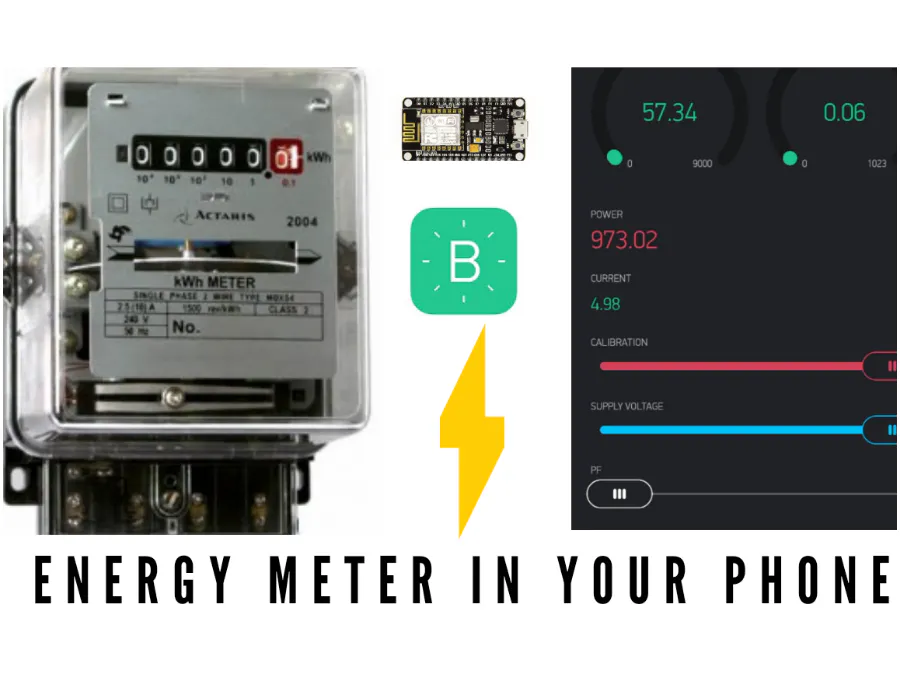## Things used in this project

### Hardware components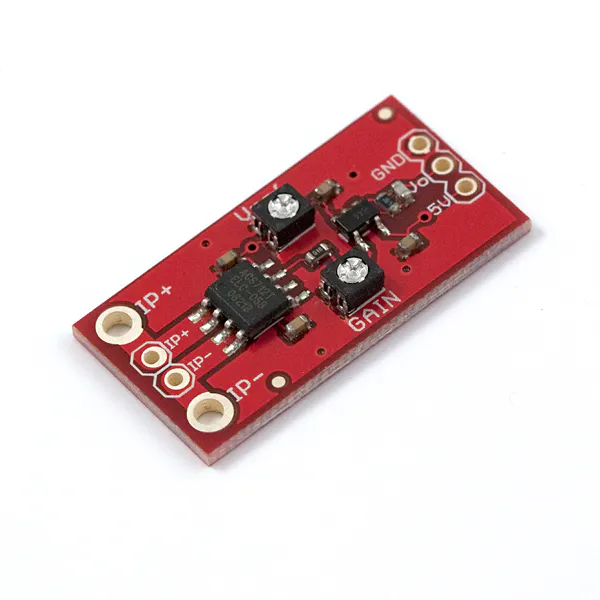SparkFun Low Current Sensor Breakout - ACS712
×1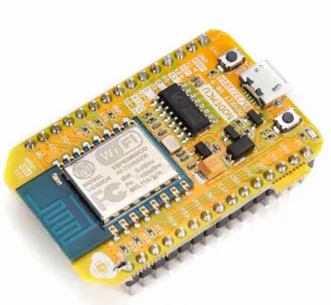NodeMCU ESP8266 Breakout Board
×1

### Software apps and online servicesArduino IDEBlynkIFTTT Maker service

### Hand tools and fabrication machines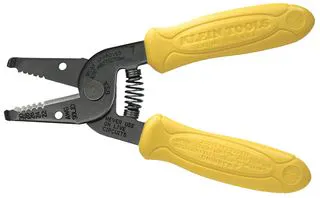Wire Stripper & Cutter, 18-10 AWG / 0.75-4mm² Capacity Wires

## Schematics

### Diagram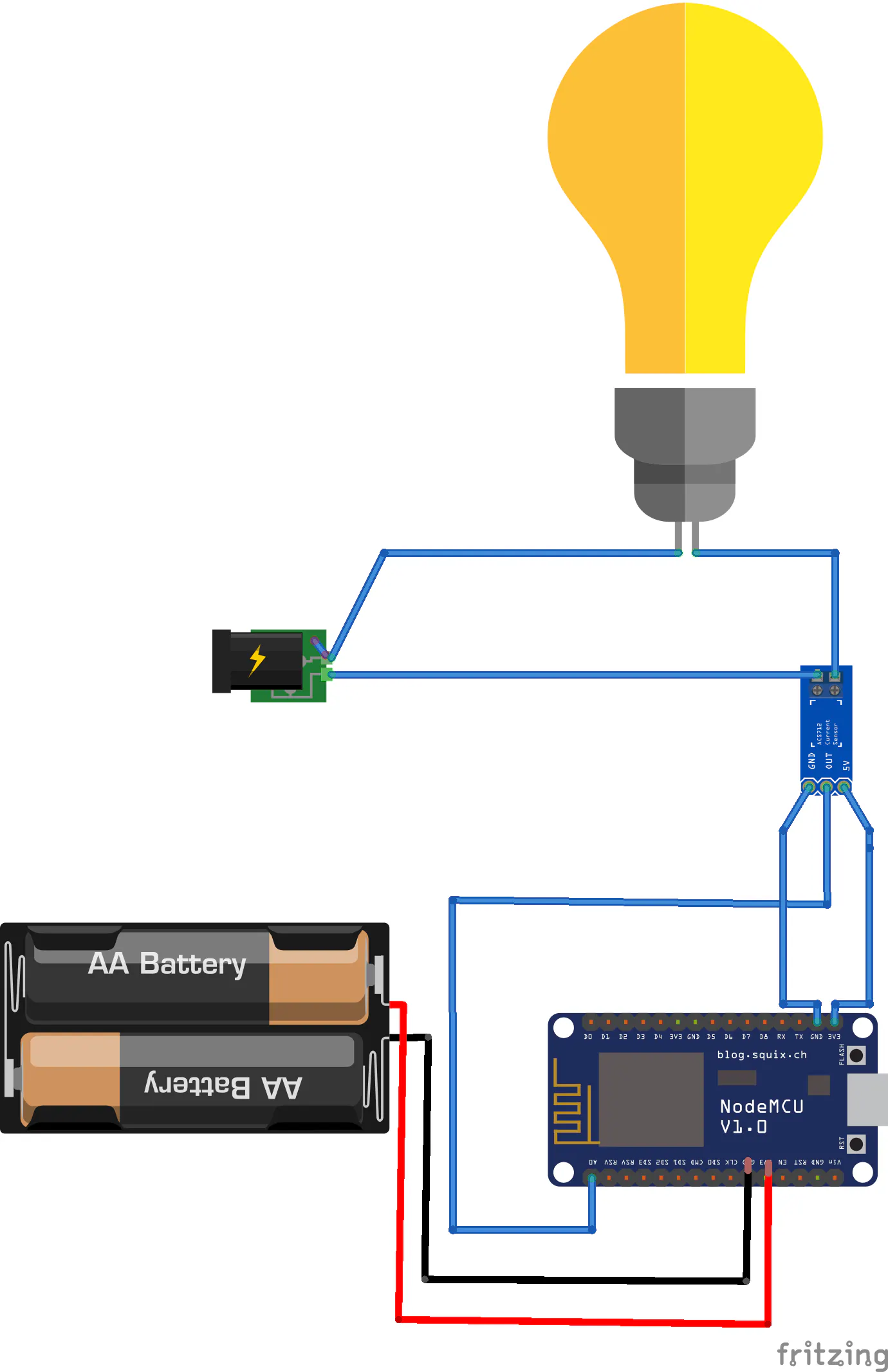## Code

### Energy meter

Arduino
```// Arduino Energy Meter V2.0
// This code is for This code is for Wemos(ESP8266) based Energy monitoring Device
// This code is a modified version of sample code from https://github.com/pieman64/ESPecoMon
// Last updated on 30.05.2018

//#define BLYNK_DEBUG
#define BLYNK_PRINT Serial

#include <ESP8266WiFi.h>
#include <BlynkSimpleEsp8266.h>

BlynkTimer timer;

char auth[] = "blynk auth key";
char ssid[] = "wifi ssid";
char server[]     = "blynk-cloud.com";    // ip or domain
char myhostname[] = "Energy-Meter-V2.0";  // for OTA and router identification

const int Sensor_Pin = A0;
unsigned int Sensitivity = 185;   // 185mV/A for 5A, 100 mV/A for 20A and 66mV/A for 30A Module
float Vpp = 0; // peak-peak voltage
float Vrms = 0; // rms voltage
float Irms = 0; // rms current
float Supply_Voltage = 233.0;           // reading from DMM
float Vcc = 5.0;         // ADC reference voltage // voltage at 5V pin
float power = 0;         // power in watt
float Wh =0 ;             // Energy in kWh
float Watt=0;
unsigned long last_time =0;
unsigned long current_time =0;
unsigned long interval = 100;
unsigned int calibration = 100;  // V2 slider calibrates this
unsigned int pF = 85;           // Power Factor default 95
float bill_amount = 0;   // 30 day cost as present energy usage incl approx PF
unsigned int energyTariff = 8.0; // Energy cost in INR per unit (kWh)

void getACS712() {  // for AC
Vpp = getVPP();
Vrms = (Vpp/2.0) *0.707;
Vrms = Vrms - (calibration / 10000.0);     // calibtrate to zero with slider
Irms = (Vrms * 1000)/Sensitivity ;
Irms=Irms+4.6;
if((Irms > -0.015) && (Irms < 0.008)){  // remove low end chatter
Irms = 0.0;
}
//Irms=Irms+3.6;
power= (Supply_Voltage * Irms) * (pF / 100.0);
//power=power+881;
last_time = current_time;
current_time = millis();
Wh = Wh+  power *(( current_time -last_time) /3600000.0) ; // calculating energy in Watt-Hour
Watt=Wh/1000;
bill_amount = Wh * (energyTariff/1000);
Serial.print("Irms:  ");
Serial.print(String(Irms, 3));
Serial.println(" A");
Serial.print("Power: ");
Serial.print(String(power, 3));
Serial.println(" W");
Serial.print("  Bill Amount: INR");
Serial.println(String(bill_amount, 2));
Blynk.virtualWrite(V0, String (Wh));
Blynk.virtualWrite(V8, String (Watt));// gauge
Blynk.virtualWrite(V1, String(bill_amount, 2));
Blynk.virtualWrite(V2, String(power,2));
Blynk.virtualWrite(V3, String(Irms, 3));
}

float getVPP()
{
float result;
int maxValue = 0;
int minValue = 1024;
uint32_t start_time = millis();
while((millis()-start_time) < 950) //read every 0.95 Sec
{
{
}
{
}
}
result = ((maxValue - minValue) * Vcc) / 1024.0;
return result;
}

BLYNK_WRITE(V4) {  // calibration slider 50 to 200
calibration = param.asInt();
}
BLYNK_WRITE(V5) {  // set supply voltage slider 70 to 260
Supply_Voltage = param.asInt();
}
BLYNK_WRITE(V6) {  // PF slider 60 to 100 i.e 0.60 to 1.00, default 85
pF = param.asInt();
}
BLYNK_WRITE(V7) {  // Energy tariff slider 1 to 20, default 8 (Rs.8.0 / kWh)
energyTariff = param.asInt();
}

void setup() {
// display.begin();
WiFi.hostname(myhostname);
Serial.begin(115200);
Serial.println("\n Rebooted");
WiFi.mode(WIFI_STA);
#ifdef CLOUD
Blynk.begin(auth, ssid, pass);
#else
Blynk.begin(auth, ssid, pass, server);
#endif
while (Blynk.connect() == false) {}
// ArduinoOTA.setHostname(myhostname);
//ArduinoOTA.begin();
timer.setInterval(2000L, getACS712); // get data every 2s
}

BLYNK_CONNECTED(){
Blynk.syncAll();
}

void loop() {

//displaydata();
Blynk.run();
//  ArduinoOTA.handle();
timer.run();

}
```

## Credits

### INSANE SCIENCE

10 projects • 3 followers
We are mechanical student, we been since 2014